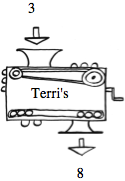Home > A2C > Chapter 1 > Lesson 1.1.2 > Problem1-15

1-15.Terri's project for the Math Fair was a magnificent black box that she called a function machine. If you put $3$ into her machine, the output would be $8$. If you put in $10$, the output would be $29$; and if you put in 20, it would be 59..

1. What would her machine do to the input $5$? What about $−1$? What about $x$? Making an input→output table may help you figure this out.

Look for a pattern. Use the eTool below.

An input of $30$ gives output $89$. Think of a number to multiply the input by.

$\left. \begin{array} { | c | c | c | c | c | c | } \hline x & { 3 } & { 10 } & { 20 } & { 5 } & { - 1 } \\ \hline 3 x - 1 & { 8 } & { 29 } & { 59 } & { 14 } & { - 4 } \\ \hline \end{array} \right.$

2. Write a rule for Terri's machine.

$(100, 299)$ could be in the table. What is each x-value multiplied by?

What is the output when $x = 0$?

If the input is $x$ what is the output? What is the equation?

$f(x) = 3x − 1$

Complete the table in the eTool below to help solve the problem.
Click the link at right for the full version of the eTool:  1-15 HW eTool